The datamanager classes and functions are useful for locating the correct data file for a particular day and manipulating data and subsets in a generic way.

Authors: Jon Niehof

Institution: University of New Hampshire

Contact: Jonathan.Niehof@unh.edu

## Examples¶

Examples go here

Classes

 `DataManager`(directories, file_fmt[, …]) THIS CLASS IS NOT YET COMPLETE, doesn’t do much useful.

Functions

 `apply_index`(data, idx) Apply an array of indices to data. `array_interleave`(array1, array2, idx) Create an array containing all elements of both array1 and array2 `axis_index`(shape[, axis]) Returns array of indices along axis, for all other axes `flatten_idx`(idx[, axis]) Convert multidimensional index into index on flattened array. `insert_fill`(times, data[, fillval, tol, …]) Populate gaps in data with fill. `rebin`(data, bindata, bins[, axis, bintype, …]) Rebin one axis of input data based on values of another array `rev_index`(idx[, axis]) From an index, return an index that reverses the action of that index `values_to_steps`(array[, axis]) Transform values along an axis to their order in a unique sequence.
class `spacepy.datamanager.``DataManager`(directories, file_fmt, descend=False, period=None)[source]

THIS CLASS IS NOT YET COMPLETE, doesn’t do much useful.

Will have to do something that allows the config file to specify regex and other things, and then just the directory to be changed (since regex, etc.

Parameters
directorieslist

A list of directories that might contain the data

file_fmtstring

Regular expression that matches the files desired. Will also recognize strftime parameters %w %d %m %y %Y %H %M %s %j %U %W, all zero-pad. https://docs.python.org/2/library/datetime.html#strftime-strptime-behavior Can have subdirectory reference, but separator should be unix-style, with no leading slash.

periodstring

Size of file; can be a number followed by one of d, m, y, H, M, s. Anything else is assumed to be “irregular” and files treated as if there are neither gaps nor overlaps in the sequence. If not specified, will be assumed to match one count of the smallest unit in the format string.

Examples
=======
`files_matching`(dt=None)[source]

Return all the files matching this file format

Parameters
dtdatetime

Optional; if specified, match only files for this date.

Returns
outgenerator

Iterates over every file matching the format specified at creation. Note this is specified in native path format!

`get_filename`(dt)[source]

Returns the filename corresponding to a particular point in time

`spacepy.datamanager.``apply_index`(data, idx)[source]

Apply an array of indices to data.

Most useful in dealing with the output from `numpy.argsort()`, and best explained by the example.

Parameters
dataarray

Input data, at least two dimensional. The 0th dimension is treated as a “time” or “record” dimension.

idxsequence

2D index to apply to the import data. The 0th dimension must be the same size as `data`’s 0th dimension. Dimension 1 must be the same size as one other dimension in data (the first match found is used); this is referred to as the “index dimension.”

Returns
datasequence

View of `data`, with index applied. For each index of the 0th dimension, the values along the index dimension are obtained by applying the value of `idx` at the same index in the 0th dimension. This is repeated across any other dimensions in `data`.

Warning

No guarantee is made whether the returned data is a copy of the input data. Modifying values in the input may change the values of the input. Call `copy()` if a copy is required.

Raises
ValueErrorif can’t match the shape of data and indices

Examples

Assume `flux` is a 3D array of fluxes, with a value for each of time, pitch angle, and energy. Assume energy is not necessarily constant in time, nor is ordered in the energy dimension. If `energy` is a 2D array of the energies as a function of energy step for each time, then the following will sort the flux at each time and pitch angle in energy order.

```>>> idx = numpy.argsort(energy, axis=1)
>>> flux_sorted = spacepy.datamanager.apply_index(flux, idx)
```
`spacepy.datamanager.``array_interleave`(array1, array2, idx)[source]

Create an array containing all elements of both array1 and array2

`idx` is an index on the output array which indicates which elements will be populated from `array1`, i.e., `out[idx] == array1` (in order.) The other elements of `out` will be filled, in order, from `array2`.

Parameters
array1array

Input data.

array2array

Input data. Must have same number of dimensions as `array1`, and all dimensions except the zeroth must also have the same length.

idxarray

A 1D array of indices on the zeroth dimension of the output array. Must have the same length as the zeroth dimension of `array1`.

Returns
outarray

All elements from `array1` and `array2`, interleaved according to `idx`.

Examples

```>>> import numpy
>>> import spacepy.datamanager
>>> a = numpy.array([10, 20, 30])
>>> b = numpy.array([1, 2])
>>> idx = numpy.array([1, 2, 4])
>>> spacepy.datamanager.array_interleave(a, b, idx)
array([ 1, 10, 20,  2, 30])
```
`spacepy.datamanager.``axis_index`(shape, axis=- 1)[source]

Returns array of indices along axis, for all other axes

Parameters
shapetuple

Shape of the output array

Returns
idxarray

An array of indices. The value of each element is that element’s index along `axis`.

Other Parameters
axisint

Axis along which to return indices, defaults to the last axis.

`numpy.mgrid`

This function is a special case

Examples

For a shape of `(i, j, k, l)` and `axis` = -1, `idx[i, j, k, :] = range(l)` for all `i`, `j`, `k`.

Similarly, for the same shape and `axis = 1`, `idx[i, :, k, l] = range(j)` for all `i`, `k`, `l`.

```>>> import numpy
>>> import spacepy.datamanager
>>> spacepy.datamanager.axis_index((5, 3))
array([[0, 1, 2],
[0, 1, 2],
[0, 1, 2],
[0, 1, 2],
[0, 1, 2]])
>>> spacepy.datamanager.axis_index((5, 3), 0)
array([[0, 0, 0],
[1, 1, 1],
[2, 2, 2],
[3, 3, 3],
[4, 4, 4]])
```
`spacepy.datamanager.``flatten_idx`(idx, axis=- 1)[source]

Convert multidimensional index into index on flattened array.

Convert a multidimensional index, that is values along a particular axis, so that it can derefence the flattened array properly. Note this is not the same as `ravel_multi_index()`.

Parameters
idxarray

Input index, i.e. a list of elements along a particular axis, in the style of `argsort()`.

Returns
flatarray

A 1D array of indices suitable for indexing the flat version of the array

Other Parameters
axisint

Axis along which `idx` operates, defaults to the last axis.

Examples

```>>> import numpy
>>> import spacepy.datamanager
>>> data = numpy.array([[3, 1, 2], [3, 2, 1]])
>>> idx = numpy.argsort(data, -1)
>>> idx_flat = spacepy.datamanager.flatten_idx(idx)
>>> data.ravel() #flat array
array([3, 1, 2, 3, 2, 1])
>>> idx_flat #indices into the flat array
array([1, 2, 0, 5, 4, 3])
>>> data.ravel()[idx_flat] #index applied to the flat array
array([1, 2, 3, 1, 2, 3])
```
`spacepy.datamanager.``insert_fill`(times, data, fillval=nan, tol=1.5, absolute=None, doTimes=True)[source]

Populate gaps in data with fill.

Continuous data are often treated differently from discontinuous data, e.g., matplotlib will draw lines connecting data points but break the line at fill. Often data will be irregularly sampled but also contain large gaps that are not explicitly marked as fill. This function adds a single record of explicit fill to each gap, defined as places where the spacing between input times is a certain multiple of the median spacing.

Parameters
timessequence

Values representing when the data were taken. Must be one-dimensional, i.e., each value must be scalar. Not modified

datasequence

Input data.

Returns
times, datatuple of sequence

Copies of input times and data, fill added in gaps (`doTimes` True)

datasequence

Copy of input data, with fill added in gaps (`doTimes` False)

Other Parameters
fillval :

Fill value, same type as `data`. Default is `numpy.nan`. If scalar, will be repeated to match the shape of `data` (minus the time axis).

Note

The default value of `nan` will not produce good results with integer input.

tolfloat

Tolerance. A single fill value is inserted between adjacent values where the spacing in `times` is strictly greater than `tol` times the median of the spacing across all `times`. The inserted time for fill is halfway between the time on each side. (Default 1.5)

absolute :

An absolute value for maximum spacing, of a type that would result from a difference in `times`. If specified, `tol` is ignored and any gap strictly larger than `absolute` will have fill inserted.

doTimesboolean

If True (default), will return a tuple of the times (with new values inserted for the fill records) and the data with new fill values. If False, will only return the data – useful for applying fill to multiple arrays of data on the same timebase.

Raises
ValueErrorif can’t identify the time axis of data

Try using `numpy.rollaxis()` to put the time axis first in both `data` and `times`.

Examples

This example shows simple hourly data with a gap, populated with fill. Note that only a single fill value is inserted, to break the sequence of valid data rather than trying to match the existing cadence.

```>>> import datetime
>>> import numpy
>>> import spacepy.datamanager
>>> t = [datetime.datetime(2012, 1, 1, 0),
datetime.datetime(2012, 1, 1, 1),
datetime.datetime(2012, 1, 1, 2),
datetime.datetime(2012, 1, 1, 5),
datetime.datetime(2012, 1, 1, 6)]
>>> temp = [30.0, 28, 27, 32, 35]
>>> filled_t, filled_temp = spacepy.datamanager.insert_fill(t, temp)
>>> filled_t
array([datetime.datetime(2012, 1, 1, 0, 0),
datetime.datetime(2012, 1, 1, 1, 0),
datetime.datetime(2012, 1, 1, 2, 0),
datetime.datetime(2012, 1, 1, 3, 30),
datetime.datetime(2012, 1, 1, 5, 0),
datetime.datetime(2012, 1, 1, 6, 0)], dtype=object)
>>> filled_temp
array([ 30.,  28.,  27.,  nan,  32.,  35.])
```

This example plots “gappy” data with and without explicit fill values.

```>>> import matplotlib.pyplot as plt
>>> import numpy
>>> import spacepy.datamanager
>>> x = numpy.append(numpy.arange(0, 6, 0.1), numpy.arange(12, 18, 0.1))
>>> y = numpy.sin(x)
>>> xf, yf = spacepy.datamanager.insert_fill(x, y)
>>> fig = plt.figure()
>>> ax0.plot(x, y)
>>> ax1.plot(xf, yf)
>>> plt.show()
````spacepy.datamanager.``rebin`(data, bindata, bins, axis=- 1, bintype='mean', weights=None, clip=False, bindatadelta=None)[source]

Rebin one axis of input data based on values of another array

This is clearest with an example. Consider a flux as a function of time, energy, and the look direction of a detector (could be multiple detectors, or spin sectors.) The flux is then 3-D, dimensioned Nt x Ne x Nl. Now consider that each look direction has an associated pitch angle that is a function of time and thus stored in an array Nt x Nl. Then this function will redimension the flux into pitch angle bins (rather than tags.)

So consider the PA bins to have dimension Np + 1 (because it represents the edges, the number of bins is one less than the dimension.) Then the output will be dimensioned Nt x Ne x Np.

`bindata` must be same or lesser dimensionality than `data`. Any axes which are present must be either of size 1, or the same size as `data`. So for `data` 100x5x20, `bindata` may be 100x5x20, or 100, or 100x1x20, but not 100x20x5. This function will insert axes of size 1 as needed to match dimensionality.

Parameters
data`ndarray`

N-dimensional array of data to be rebinned. `nan` are ignored.

bindata`ndarray`

M-dimensional (M<=N) array of values to be compared to the bins.

bins`ndarray`

1-D array of bin edges. Output dimension will be this size minus 1. Any values in `bindata` that don’t fall in the bins will be omitted from the output. (See `clip` to change this behavior).

Returns
`ndarray`

`data` with one axis redimensioned, from its original dimension to the bin dimension.

Other Parameters
axisint

Axis of data to rebin. This axis will disappear in the output and be replaced with an axis of the size of `bins` less one. (Default -1, last axis)

bintypestr
Type of rebinning to perform:
mean

Return the mean of all values in the bin (default)

unc

Return the quadrature mean of all values in the bin, for propagating uncertainty

count

Return the count of values that fall in each bin.

weights`ndarray`

Relative weight of each sample in `bindata`. Must be same shape as `bindata`. Purely relative, i.e. the output is only affected based on the total of `weights` if `bintype` is `count`. Note if `weights` is specified, `count` returns the sum of the weights, not the count of individual samples.

clipboolean

Clip data to the bins. If true, all input data will be assigned a bin and data outside the range of the bin edges will be assigned to the extreme bins. If false (default), input data outside the bin ranges will be ignored.

bindatadelta`ndarray`

By default, the `bindata` are treated as point values. If `bindatadelta` is specified, it is treated as the half-width of the `bindata`, allowing a single input value to be split between output bins. Must be scalar, or same shape as bindata. Note that input values are not weighted by the bin width, but by number of input values or by `weights`. (Combining `weights` with `bindatadelta` is not comprehensively tested.)

Examples

Consider a particle flux distribution that’s a function of energy and pitch angle. For simplicity, assume that the energy dependence is a simple power law and the pitch angle dependence is Gaussian, with a peak whose position oscillates in time over a period of about one hour. This is fairly non-physical but illustrative.

First making the relevant imports:

```>>> import matplotlib.pyplot
>>> import numpy
>>> import spacepy.datamanager
>>> import spacepy.plot
```

The functional form of the flux is then:

```>>> def j(e, t, a):
...     return e ** -2 * (1 / (90 * numpy.sqrt(2 * numpy.pi))) \
...     * numpy.exp(
...         -0.5 * ((a - 90 + 90 * numpy.sin(t / 573.)) / 90.) ** 2)
```

Illustrating the flux at one energy as a function of pitch angle:

```>>> times = numpy.arange(0., 7200, 5)
>>> alpha = numpy.arange(0, 181., 2)
# Add a dimension so the flux is a 2D array
>>> flux = j(1., numpy.expand_dims(times, 1),
...          numpy.expand_dims(alpha, 0))
>>> spacepy.plot.simpleSpectrogram(times, alpha, flux, cb=False,
...                                ylog=False)
>>> matplotlib.pyplot.ylabel('Pitch angle (deg)')
>>> matplotlib.pyplot.xlabel('Time (sec)')
>>> matplotlib.pyplot.title('Flux at 1 MeV')
```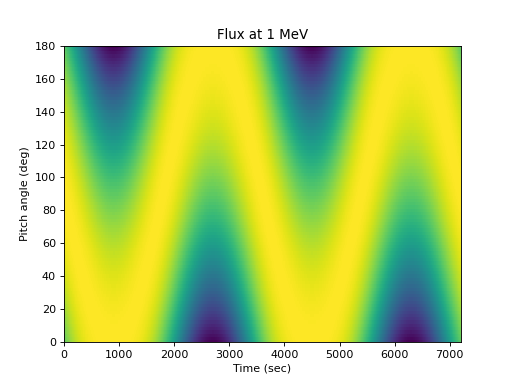Or the flux at one pitch angle as a function of energy:

```>>> energies = numpy.logspace(0, 3, 50)
>>> flux = j(numpy.expand_dims(energies, 0),
...          numpy.expand_dims(times, 1), 90.)
>>> spacepy.plot.simpleSpectrogram(times, energies, flux, cb=False)
>>> matplotlib.pyplot.ylabel('Energy (MeV)')
>>> matplotlib.pyplot.xlabel('Time (sec)')
>>> matplotlib.pyplot.title('Flux at 90 degrees')
```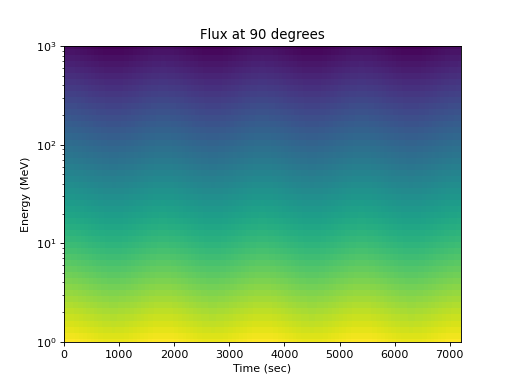The measurement is usually not aligned with a pitch angle grid, and the detector pointing in pitch angle space usually varies with time. Taking a very simple case of eight detectors that sweep through pitch angle space in an organized fashion at ten degrees per minute, the measured pitch angle as a function of detector and time is:

```>>> def pa(d, t):
...     return (d * 22.5 + t * (2 * (d % 2) - 1)) % 180
>>> lines = matplotlib.pyplot.plot(
...     times, pa(numpy.arange(8).reshape(1, -1), times.reshape(-1, 1)),
...     marker='o', ms=1, linestyle='')
>>> matplotlib.pyplot.legend(lines,
...     ['Detector {}'.format(i) for i in range(4)], loc='best')
>>> matplotlib.pyplot.xlabel('Time (sec)')
>>> matplotlib.pyplot.ylabel('Pitch angle (deg)')
>>> matplotlib.pyplot.title('Measured pitch angle by detector')
```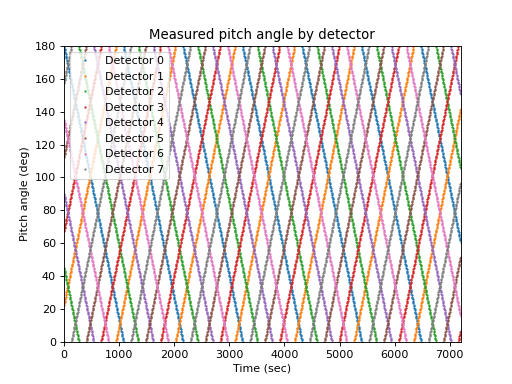Assuming a coarser measurement in time and energy than used to illustrate the distribution above, the measured flux as a function of time, detector, and energy is constructed:

```>>> times = numpy.arange(0., 7200, 300) #5 min cadence
>>> alpha = pa(numpy.arange(8).reshape(1, -1), times.reshape(-1, 1))
>>> energies = numpy.logspace(0, 3, 10) #10 energy channels (3/decade)
# Every dimension (t, detector, e) gets its own numpy axis
>>> flux = j(numpy.reshape(energies, (1, 1, -1)),
numpy.reshape(times, (-1, 1, 1)),
numpy.expand_dims(alpha, -1))
>>> flux.shape
(24, 8, 10)
```

The flux at an energy as a function of detector is not very useful:

```>>> spacepy.plot.simpleSpectrogram(times, numpy.arange(8),
...                                flux[..., 0], cb=False, ylog=False)
>>> matplotlib.pyplot.ylabel('Detector')
>>> matplotlib.pyplot.xlabel('Time (sec)')
>>> matplotlib.pyplot.title('Flux at 1 MeV')
```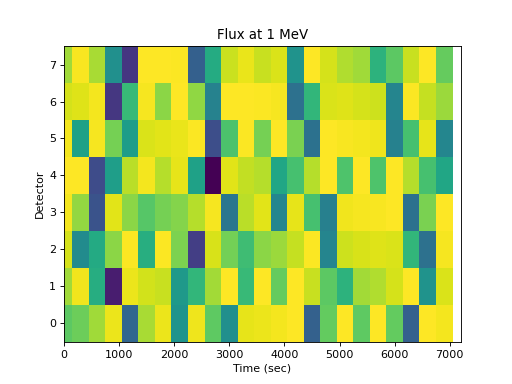As a function of energy for one detector, the energy dependence is apparent but time and pitch angle effects are confounded:

```>>> spacepy.plot.simpleSpectrogram(times, energies, flux[:, 0, :],
...                                cb=False)
>>> matplotlib.pyplot.ylabel('Energy (MeV)')
>>> matplotlib.pyplot.xlabel('Time (sec)')
>>> matplotlib.pyplot.title('Flux in detector 0')
```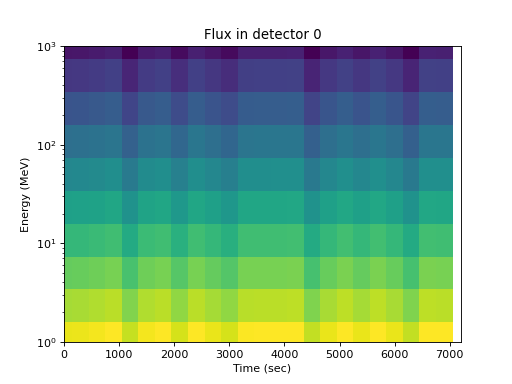What is needed is to recover the array of flux dimensioned by time, pitch angle, and energy, with appropriate pitch angle bins. The assumption is that the pitch angle as a function of time and detector is measured and thus the `alpha` array is available. Using that array, `rebin` can change flux from time, detector, energy bins to time, pitch angle, energy bins. The axis 1 changes from a detector dimension to pitch angle:

```>>> pa_bins = numpy.arange(0, 181, 36)
>>> flux_by_pa = spacepy.datamanager.rebin(
...     flux, alpha, pa_bins, axis=1)
>>> flux_by_pa.shape
(24, 6, 10)
```

This can then be visualized. The pitch angle coverage is not perfect, but the original shape of the distribution is apparent, and further analysis can be performed on the regular pitch angle grid:

```>>> spacepy.plot.simpleSpectrogram(times, pa_bins, flux_by_pa[..., 0],
...                                cb=False, ylog=False)
>>> matplotlib.pyplot.ylabel('Pitch angle (deg)')
>>> matplotlib.pyplot.xlabel('Time (sec)')
>>> matplotlib.pyplot.title('Flux at 1MeV')
```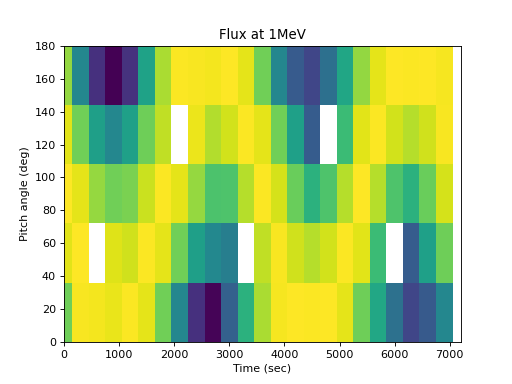Or by energy:

```>>> spacepy.plot.simpleSpectrogram(times, energies, flux_by_pa[:, 2, :],
...                                cb=False)
>>> matplotlib.pyplot.ylabel('Energy (MeV)')
>>> matplotlib.pyplot.xlabel('Time (sec)')
>>> matplotlib.pyplot.title('Flux at 90 degrees')
```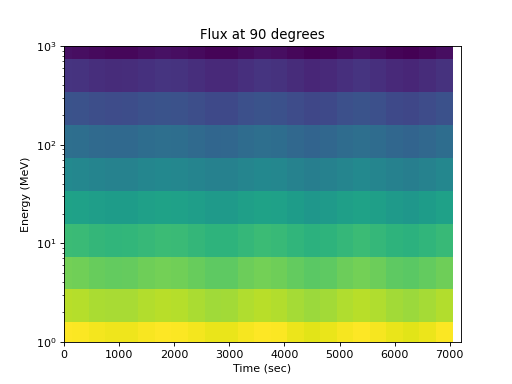`rebin` can be used for higher dimension data, if the pitch angle itself depends on energy (e.g. if an energy sweep takes substantial time), and to propagate uncertainties through the rebinning. It can also be used to rebin on the time axis, e.g. for transforming time base.

`spacepy.datamanager.``rev_index`(idx, axis=- 1)[source]

From an index, return an index that reverses the action of that index

Essentially, `a[idx][rev_index(idx)] == a`

Note

This becomes more complicated in multiple dimensions, due to the vagaries of applying a multidimensional index.

Parameters
idxarray

Indices onto an array, often the output of `argsort()`.

Returns
rev_idxarray

Indices that, when applied to an array after `idx`, will return the original array (before the application of `idx`).

Other Parameters
axisint

Axis along which to return indices, defaults to the last axis.

Examples

```>>> import numpy
>>> import spacepy.datamanager
>>> data = numpy.array([7, 2, 4, 6, 3])
>>> idx = numpy.argsort(data)
>>> data[idx] #sorted
array([2, 3, 4, 6, 7])
>>> data[idx][spacepy.datamanager.rev_index(idx)] #original
array([7, 2, 4, 6, 3])
```
`spacepy.datamanager.``values_to_steps`(array, axis=- 1)[source]

Transform values along an axis to their order in a unique sequence.

Useful in, e.g., converting a list of energies to their steps.

Parameters
arrayarray

Input data.

Returns
stepsarray

An array, the same size as `array`, with values along `axis` corresponding to the position of the value in `array` in a unique, sorted, set of the values in `array` along that axis. Differs from `argsort()` in that identical values will have identical step numbers in the output.

Other Parameters
axisint

Axis along which to find the steps.

Examples

```>>> import numpy
>>> import spacepy.datamanager
>>> data = [[10., 12., 11., 9., 10., 12., 11., 9.],
[10., 12., 11., 9., 14., 16., 15., 13.]]
>>> spacepy.datamanager.values_to_steps(data)
array([[1, 3, 2, 0, 1, 3, 2, 0],
[1, 3, 2, 0, 5, 7, 6, 4]])
```